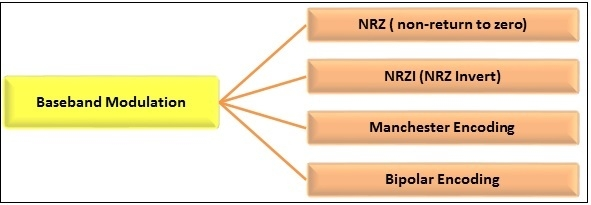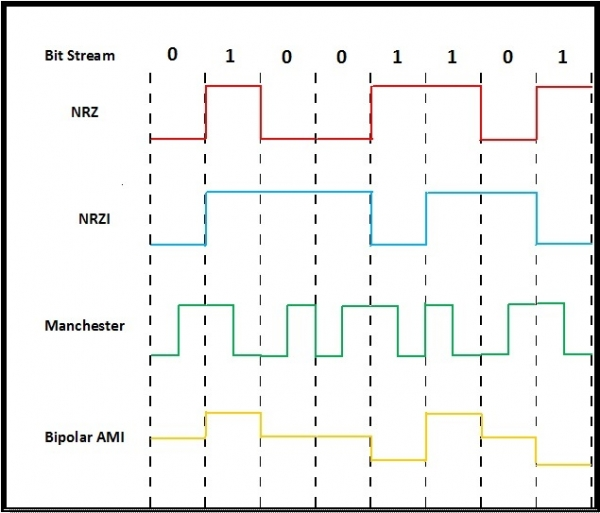# Baseband Transmission

In baseband transmission, the data bits are directly converted into signals. Generally a higher voltage level represents the bit 1, while a lower voltage level represents bit 0.

The different encoding schemes are shown in the diagram. Among these, the first three are come in the category of polar encoding. In polar signaling, one logical state is represented by only one voltage state. In bipolar schemes, two voltage levels may be used to represent a logical state.NRZ is an unipolar coding scheme. Here, a high voltage represents 1, while a low voltage represents 0. Non-return to zero implies that the signal does not return to zero at the middle of the bit.

## NRZ-I (NRZ Invert)

NRZ-I is an polar coding scheme. In NRZI, bit 1 is represented by a transition in voltage, while bit 0 is represented by no such transitions. It has an average signal rate of N/2 baud.

## Manchester Encoding

Manchester encoding is a biphase coding scheme. Bit 1 is represented by a voltage transition from high to low, while bit 0 is represented by a voltage transition from low to high.

## Bipolar Encoding

It is also called Alternate Mark Inversion or AMI. Three voltage levels are used here. Here, bit 0 is represented by no line signal, while bit 1 is represented by a positive or negative voltage level, alternating for successive ones.

### Example

Let there be a bit stream 01001101. The following diagram plots the different encoding schemes −Updated on: 03-Aug-2019

3K+ Views